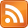# kaashif's blog

Programming, with some mathematics on the side## Writing a parser for a function call is surprisingly hard

### 2020-05-17

I was recently trying to get to grips with the GAP programming language. For those not familiar, it's the programming language for the GAP computational algebra system. It has tons of algorithms implemented for group theory, representation theory, algebraic number theory, and so on. I was thinking about implementing a TypeScript-style transpiler so I could program with some types, and the first step is to parse the syntax.

To get the most elegant parser, I went for a parser written in Haskell using Parsec, which is an elegant library for LL(1) parsers.

The first problem I ran into was that GAP supports several function call syntaxes:

f(x, y, z); # positional
g(p := 1, q := 2); # named-ish parameters
h(x, y, z : p := 1); # mixed


This is surprisingly non-trivial to parse in general! The path is fraught with infinite recursion, ambiguities, and backtracking.

## First problem: infinite recursion

First, we need to understand how Parsec works, it's an LL(1) parser. That means it can be used to parse context-free languages, it tries to perform a leftmost derivation, with 1 token of lookahead. It's easier to explain this with an example. I'll use the notation of production rules for formal languages.

We want to say that a function call is some expression, with a parenthesis delimited argument list which is how we tell we're calling something. So we have things like f(x) but also f(x)(y) which is calling the result of f(x) with the argument y. Using start symbol $E$ (for "expression"), the function call grammar looks like:

\begin{align} E &\to E(A) \\ E &\to L \\ A &\to E, A \\ A &\to E \end{align}

where $L$ can be any literal, and $A$ represents an argument list.

We'd write this in Parsec as something like:

data Expr = Variable String | FunctionCall Expr [Expr]

expression = functionCall <|> (Variable <$> identifier) functionCall = FunctionCall <$> expression <*> (parens commaSep expression)  where we're parsing a string into the data type Expr. The parameters to FuncCall are: the expression we're calling, and the argument list. This is a cut down example, so I say we only have variables as the literals. The problem comes when we try to run this on a string: parse expression "" "f(x)"  This will just hang at 100% CPU usage. Why? Because the definition of an expression is immediately left-recursive. Parsec is LL(1) so tries to recurse as far as possible on the left when parsing, and we can just keep going: try to parse an expression, the first step is to check if it's a function call. To check if it's a function call, we need to parse an expression, the first step to that is check if it's a function call, and so on. What's the solution? We can cleverly transform the grammar to avoid the immediate left recursion. When we actually see a function expression, what do we see? A non-function call expression on the far left, then a lot of argument lists. As production rules: \begin{align} E &\to LM \\ E &\to L \\ M &\to M(A) \\ M &\to (A) \end{align} whereA$is the same as before. In Parsec: expression = functionCall <|> (Variable <$> identifier)
functionCall = foldl' FuncCall <$> (Variable <$> identifier) <*> many1 argList
where argList = parens $commaSep expression  Some explanation: if we have a string "f(x)(y)" this is parsed as: • Variable "f" when we see the f • FuncCall (Variable "f") [Variable "x"] when we see the argument list (x). • FuncCall (FuncCall (Variable "f") [Variable "x"]) [Variable "y"] when we see the argument list (y). Perfect! This avoids the infinite recursion. Next problem! ## Horrific named argument grammar To implement the keyword arguments, we need to make a few changes to the argList parser. The obvious thing to do is just implement the alternatives in a big list: argList :: Parser ([Expr], [(String, Expr)]) argList = parens$ do
args <- commaSep expression
if length args > 0
then colon
else return ()
named <- commaSep namedArg
where namedArg = (,) <$> identifier <* reservedOp ":=" <*> expression  where argList now returns a tuple of the non-named arguments and the named arguments. But this doesn't work! How do you parse the following argument list: (x := 1)  The "correct" parser would see this as a single named argument, but our parser currently sees this as a variable x, then the colon separating the non-named from named arguments, then an =. That's a syntax error - the parser fails! The solution is to try the cases in order, explicitly. argList :: Parser ([Expr], [(String, Expr)]) argList = parens$ try justNamed <|> try both <|> justNotNamed
where
justNamed = (,) [] $commaSep1 namedArg namedArg = (,) <$> identifier <* reservedOp ":=" <*> expression
justNotNamed = (,) <\$> commaSep expression <*> pure []
both = do
notNamed <- justNotNamed
colon
named <- justNamed
return (fst notNamed, snd named)


Ah, there we go.

## Conclusion

Parsing is non-trivial, surprisingly. To see my code in action, check out the full source of my parser for GAP (not yet fully tested).

Please don't complain that the code in this post doesn't work, look at that repo for the real working source!